Linear Regression and Correlation

# 74 How to Use Microsoft Excel® for Regression Analysis

This section of this chapter is here in recognition that what we are now asking requires much more than a quick calculation of a ratio or a square root. Indeed, the use of regression analysis was almost non- existent before the middle of the last century and did not really become a widely used tool until perhaps the late 1960’s and early 1970’s. Even then the computational ability of even the largest IBM machines is laughable by today’s standards. In the early days programs were developed by the researchers and shared. There was no market for something called “software” and certainly nothing called “apps”, an entrant into the market only a few years old.

With the advent of the personal computer and the explosion of a vital software market we have a number of regression and statistical analysis packages to choose from. Each has their merits. We have chosen Microsoft Excel because of the wide-spread availability both on college campuses and in the post-college market place. Stata is an alternative and has features that will be important for more advanced econometrics study if you choose to follow this path. Even more advanced packages exist, but typically require the analyst to do some significant amount of programing to conduct their analysis. The goal of this section is to demonstrate how to use Excel to run a regression and then to do so with an example of a simple version of a demand curve.

The first step to doing a regression using Excel is to load the program into your computer. If you have Excel you have the Analysis ToolPak although you may not have it activated. The program calls upon a significant amount of space so is not loaded automatically.

To activate the Analysis ToolPak follow these steps:

Click “File” > “Options” > “Add-ins” to bring up a menu of the add-in “ToolPaks”. Select “Analysis ToolPak” and click “GO” next to “Manage: excel add-ins” near the bottom of the window. This will open a new window where you click “Analysis ToolPak” (make sure there is a green check mark in the box) and then click “OK”. Now there should be an Analysis tab under the data menu. These steps are presented in the following screen shots.Click “Data” then “Data Analysis” and then click “Regression” and “OK”. Congratulations, you have made it to the regression window. The window asks for your inputs. Clicking the box next to the Y and X ranges will allow you to use the click and drag feature of Excel to select your input ranges. Excel has one odd quirk and that is the click and drop feature requires that the independent variables, the X variables, are all together, meaning that they form a single matrix. If your data are set up with the Y variable between two columns of X variables Excel will not allow you to use click and drag. As an example, say Column A and Column C are independent variables and Column B is the Y variable, the dependent variable. Excel will not allow you to click and drop the data ranges. The solution is to move the column with the Y variable to column A and then you can click and drag. The same problem arises again if you want to run the regression with only some of the X variables. You will need to set up the matrix so all the X variables you wish to regress are in a tightly formed matrix. These steps are presented in the following scene shots.Once you have selected the data for your regression analysis and told Excel which one is the dependent variable (Y) and which ones are the independent valuables (X‘s), you have several choices as to the parameters and how the output will be displayed. Refer to screen shot (Figure) under “Input” section. If you check the “labels” box the program will place the entry in the first column of each variable as its name in the output. You can enter an actual name, such as price or income in a demand analysis, in row one of the Excel spreadsheet for each variable and it will be displayed in the output.

The level of significance can also be set by the analyst. This will not change the calculated t statistic, called t stat, but will alter the p value for the calculated t statistic. It will also alter the boundaries of the confidence intervals for the coefficients. A 95 percent confidence interval is always presented, but with a change in this you will also get other levels of confidence for the intervals.

Excel also will allow you to suppress the intercept. This forces the regression program to minimize the residual sum of squares under the condition that the estimated line must go through the origin. This is done in cases where there is no meaning in the model at some value other than zero, zero for the start of the line. An example is an economic production function that is a relationship between the number of units of an input, say hours of labor, and output. There is no meaning of positive output with zero workers.

Once the data are entered and the choices are made click OK and the results will be sent to a separate new worksheet by default. The output from Excel is presented in a way typical of other regression package programs. The first block of information gives the overall statistics of the regression: Multiple R, R Squared, and the R squared adjusted for degrees of freedom, which is the one you want to report. You also get the Standard error (of the estimate) and the number of observations in the regression.

The second block of information is titled ANOVA which stands for Analysis of Variance. Our interest in this section is the column marked F. This is the calculated F statistics for the null hypothesis that all of the coefficients are equal to zero verse the alternative that at least one of the coefficients are not equal to zero. This hypothesis test was presented in 13.4 under “How Good is the Equation?” The next column gives the p value for this test under the title “Significance F”. If the p value is less than say 0.05 (the calculated F statistic is in the tail) we can say with 90 % confidence that we cannot accept the null hypotheses that all the coefficients are equal to zero. This is a good thing: it means that at least one of the coefficients is significantly different from zero thus do have an effect on the value of Y.

The last block of information contains the hypothesis tests for the individual coefficient. The estimated coefficients, the intercept and the slopes, are first listed and then each standard error (of the estimated coefficient) followed by the t stat (calculated student’s t statistic for the null hypothesis that the coefficient is equal to zero). We compare the t stat and the critical value of the student’s t, dependent on the degrees of freedom, and determine if we have enough evidence to reject the null that the variable has no effect on Y. Remember that we have set up the null hypothesis as the status quo and our claim that we know what caused the Y to change is in the alternative hypothesis. We want to reject the status quo and substitute our version of the world, the alternative hypothesis. The next column contains the p values for this hypothesis test followed by the estimated upper and lower bound of the confidence interval of the estimated slope parameter for various levels of confidence set by us at the beginning.

### Estimating the Demand for Roses

Here is an example of using the Excel program to run a regression for a particular specific case: estimating the demand for roses. We are trying to estimate a demand curve, which from economic theory we expect certain variables affect how much of a good we buy. The relationship between the price of a good and the quantity demanded is the demand curve. Beyond that we have the demand function that includes other relevant variables: a person’s income, the price of substitute goods, and perhaps other variables such as season of the year or the price of complimentary goods. Quantity demanded will be our Y variable, and Price of roses, Price of carnations and Income will be our independent variables, the X variables.

For all of these variables theory tells us the expected relationship. For the price of the good in question, roses, theory predicts an inverse relationship, the negatively sloped demand curve. Theory also predicts the relationship between the quantity demanded of one good, here roses, and the price of a substitute, carnations in this example. Theory predicts that this should be a positive or direct relationship; as the price of the substitute falls we substitute away from roses to the cheaper substitute, carnations. A reduction in the price of the substitute generates a reduction in demand for the good being analyzed, roses here. Reduction generates reduction is a positive relationship. For normal goods, theory also predicts a positive relationship; as our incomes rise we buy more of the good, roses. We expect these results because that is what is predicted by a hundred years of economic theory and research. Essentially we are testing these century-old hypotheses. The data gathered was determined by the model that is being tested. This should always be the case. One is not doing inferential statistics by throwing a mountain of data into a computer and asking the machine for a theory. Theory first, test follows.

These data here are national average prices and income is the nation’s per capita personal income. Quantity demanded is total national annual sales of roses. These are annual time series data; we are tracking the rose market for the United States from 1984-2017, 33 observations.

Because of the quirky way Excel requires how the data are entered into the regression package it is best to have the independent variables, price of roses, price of carnations and income next to each other on the spreadsheet. Once your data are entered into the spreadsheet it is always good to look at the data. Examine the range, the means and the standard deviations. Use your understanding of descriptive statistics from the very first part of this course. In large data sets you will not be able to “scan” the data. The Analysis ToolPac makes it easy to get the range, mean, standard deviations and other parameters of the distributions. You can also quickly get the correlations among the variables. Examine for outliers. Review the history. Did something happen? Was here a labor strike, change in import fees, something that makes these observations unusual? Do not take the data without question. There may have been a typo somewhere, who knows without review.

Go to the regression window, enter the data and select 95% confidence level and click “OK”. You can include the labels in the input range if you have put a title at the top of each column, but be sure to click the “labels” box on the main regression page if you do.

The regression output should show up automatically on a new worksheet.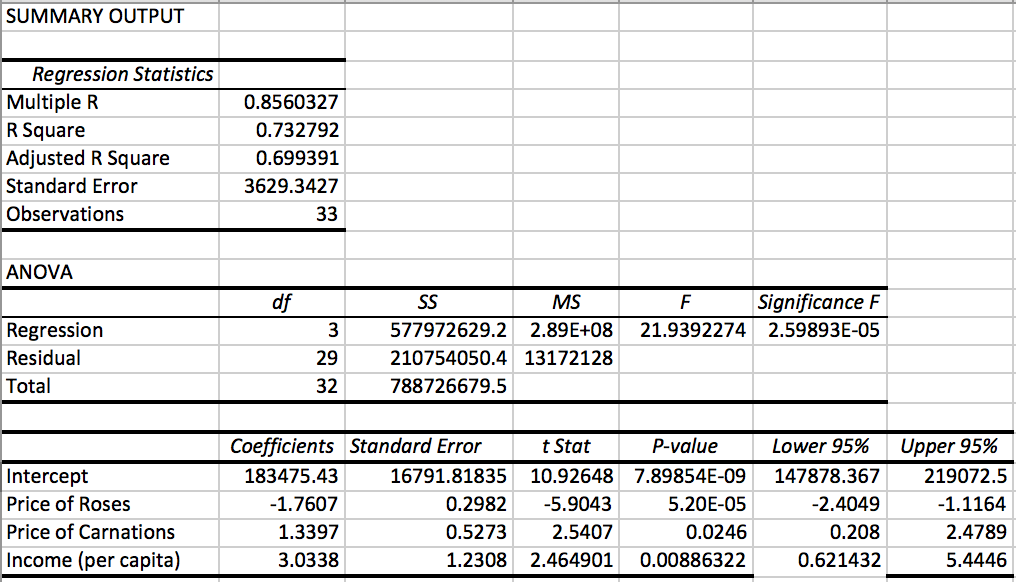The first results presented is the R-Square, a measure of the strength of the correlation between Y and X1, X2, and X3 taken as a group. Our R-square here of 0.699, adjusted for degrees of freedom, means that 70% of the variation in Y, demand for roses, can be explained by variations in X1, X2, and X3, Price of roses, Price of carnations and Income. There is no statistical test to determine the “significance” of an R2. Of course a higher R2 is preferred, but it is really the significance of the coefficients that will determine the value of the theory being tested and which will become part of any policy discussion if they are demonstrated to be significantly different form zero.

Looking at the third panel of output we can write the equation as: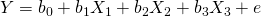where b0 is the intercept, b1 is the estimated coefficient on price of roses, and b2 is the estimated coefficient on price of carnations, b3 is the estimated effect of income and e is the error term. The equation is written in Roman letters indicating that these are the estimated values and not the population parameters, β’s.

Our estimated equation is: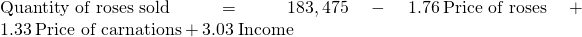We first observe that the signs of the coefficients are as expected from theory. The demand curve is downward sloping with the negative sign for the price of roses. Further the signs of both the price of carnations and income coefficients are positive as would be expected from economic theory.

Interpreting the coefficients can tell us the magnitude of the impact of a change in each variable on the demand for roses. It is the ability to do this which makes regression analysis such a valuable tool. The estimated coefficients tell us that an increase the price of roses by one dollar will lead to a 1.76 reduction in the number roses purchased. The price of carnations seems to play an important role in the demand for roses as we see that increasing the price of carnations by one dollar would increase the demand for roses by 1.33 units as consumers would substitute away from the now more expensive carnations. Similarly, increasing per capita income by one dollar will lead to a 3.03 unit increase in roses purchased.

These results are in line with the predictions of economics theory with respect to all three variables included in this estimate of the demand for roses. It is important to have a theory first that predicts the significance or at least the direction of the coefficients. Without a theory to test, this research tool is not much more helpful than the correlation coefficients we learned about earlier.

We cannot stop there, however. We need to first check whether our coefficients are statistically significant from zero. We set up a hypothesis of: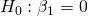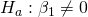for all three coefficients in the regression. Recall from earlier that we will not be able to definitively say that our estimated b1 is the actual real population of β1, but rather only that with (1-α)% level of confidence that we cannot reject the null hypothesis that our estimated β1 is significantly different from zero. The analyst is making a claim that the price of roses causes an impact on quantity demanded. Indeed, that each of the included variables has an impact on the quantity of roses demanded. The claim is therefore in the alternative hypotheses. It will take a very large probability, 0.95 in this case, to overthrow the null hypothesis, the status quo, that β = 0. In all regression hypothesis tests the claim is in the alternative and the claim is that the theory has found a variable that has a significant impact on the Y variable.

The test statistic for this hypothesis follows the familiar standardizing formula which counts the number of standard deviations, t, that the estimated value of the parameter, b1, is away from the hypothesized value, β0, which is zero in this case: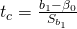The computer calculates this test statistic and presents it as “t stat”. You can find this value to the right of the standard error of the coefficient estimate. The standard error of the coefficient for b1 is Sb1 in the formula. To reach a conclusion we compare this test statistic with the critical value of the student’s t at degrees of freedom n-3-1 =29, and alpha = 0.025 (5% significance level for a two-tailed test). Our t stat for b1 is approximately 5.90 which is greater than 1.96 (the critical value we looked up in the t-table), so we cannot accept our null hypotheses of no effect. We conclude that Price has a significant effect because the calculated t value is in the tail. We conduct the same test for b2 and b3. For each variable, we find that we cannot accept the null hypothesis of no relationship because the calculated t-statistics are in the tail for each case, that is, greater than the critical value. All variables in this regression have been determined to have a significant effect on the demand for roses.

These tests tell us whether or not an individual coefficient is significantly different from zero, but does not address the overall quality of the model. We have seen that the R squared adjusted for degrees of freedom indicates this model with these three variables explains 70% of the variation in quantity of roses demanded. We can also conduct a second test of the model taken as a whole. This is the F test presented in section 13.4 of this chapter. Because this is a multiple regression (more than one X), we use the F-test to determine if our coefficients collectively affect Y. The hypothesis is: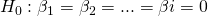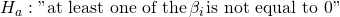Under the ANOVA section of the output we find the calculated F statistic for this hypotheses. For this example the F statistic is 21.9. Again, comparing the calculated F statistic with the critical value given our desired level of significance and the degrees of freedom will allow us to reach a conclusion.

The best way to reach a conclusion for this statistical test is to use the p-value comparison rule. The p-value is the area in the tail, given the calculated F statistic. In essence the computer is finding the F value in the table for us and calculating the p-value. In the Summary Output under “significance F” is this probability. For this example, it is calculated to be 2.6 x 10-5, or 2.6 then moving the decimal five places to the left. (.000026) This is an almost infinitesimal level of probability and is certainly less than our alpha level of .05 for a 5 percent level of significance.

By not being able to accept the null hypotheses we conclude that this specification of this model has validity because at least one of the estimated coefficients is significantly different from zero. Since F-calculated is greater than F-critical, we cannot accept H0, meaning that X1, X2 and X3together has a significant effect on Y.

The development of computing machinery and the software useful for academic and business research has made it possible to answer questions that just a few years ago we could not even formulate. Data is available in electronic format and can be moved into place for analysis in ways and at speeds that were unimaginable a decade ago. The sheer magnitude of data sets that can today be used for research and analysis gives us a higher quality of results than in days past. Even with only an Excel spreadsheet we can conduct very high level research. This section gives you the tools to conduct some of this very interesting research with the only limit being your imagination.

A computer program for multiple regression has been used to fit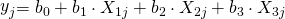.

Part of the computer output includes:

 i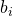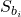0 8 1.6 1 2.2 .24 2 -.72 .32 3 0.005 0.002
1. Calculation of confidence interval for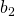consists of _______± (a student’s t value) (_______)
2. The confidence level for this interval is reflected in the value used for _______.
3. The degrees of freedom available for estimating the variance are directly concerned with the value used for _______
1. −.72, .32
2. the t value
3. the t value

An investigator has used a multiple regression program on 20 data points to obtain a regression equation with 3 variables. Part of the computer output is:

 Variable Coefficient Standard Error of1 0.45 0.21 2 0.80 0.10 3 3.10 0.86
1. 0.80 is an estimate of ___________.
2. 0.10 is an estimate of ___________.
3. Assuming the responses satisfy the normality assumption, we can be 95% confident that the value of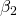is in the interval,_______ ± [t.025 ⋅ _______], where t.025 is the critical value of the student’s t distribution with ____ degrees of freedom.
1. The population value for, the change that occurs in Y with a unit change in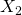, when the other variables are held constant.
2. The population value for the standard error of the distribution of estimates of.
3. .8, .1, 16 = 20 − 4.